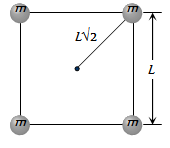Four particles each of mass M, are located at the vertices of a square with side L. The gravitational potential due to this at the centre of the square is

(a) $-\sqrt{32}\frac{GM}{L}$                                   (b) $-\sqrt{64}\frac{GM}{{L}^{2}}$

(c) zero                                              (d) $\sqrt{32}\frac{GM}{L}$

Concept Videos :-

#16 | Gravitational Potential
#17 | Expression for Gravitational Potential
#18 | Gravitational Potential due to Ring & Shell
#19 | Gravitational Potential due to Solid Sphere
#20 | Problem on Gravitational Potential

Concept Questions :-

Gravitational PotentialDifficulty Level:

• 43%
• 13%
• 40%
• 7%